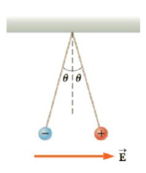Chapter 15, Problem 63AP

Chapter
Section
Textbook Problem

Two 2.0-g spheres are suspended by 10.0-cm-long light strings (Fig. P15.63). A uniform electric field is applied in the x-direction. If the spheres have charges of −5.0 × 10−8 C and +5.0 × 10−8 C, determine the electric field intensity that enables the spheres to be in equilibrium at θ = 10.To determine
The electric field intensity.

Explanation

Given info: The mass of the sphere is 2.0 g. The magnitude of charge on the spheres is 5.00×108C .

The free body diagram is,

• F1 is the force due to negatively charged sphere.
• F2 is the force exerted by the field.
• T is the tension.
• m is the mass of the ball.
• g is the acceleration due to gravity.

From the diagram,

Tcos10ο=mg

Tsin10ο+F1=F2

Dividing the 2nd equation by the 1st,

F2=F1+mgtan10ο

Formula to calculate the electric force exerted by the field is,

F2=qE

• E is the electric field.
• q is the charge on the sphere.

Formula to calculate the electric force due to negatively charged sphere is,

F1=keq2r2

• ke is the Coulomb constant.
• r is the distance between the spheres.

The distance between the spheres is,

r=2Lsin10ο

Therefore,

qE=keq(2Lsin10ο)2+mgtan10ο

On Re-arranging,

E=keq(2Lsin10ο)2+mgtan10οq

Substitute 9

Still sussing out bartleby?

Check out a sample textbook solution.

See a sample solution

The Solution to Your Study Problems

Bartleby provides explanations to thousands of textbook problems written by our experts, many with advanced degrees!

Get Started